Next: Developing Topology and Parameter Up: Parameterization Tutorial Previous: Testing out your new

# The CHARMM Force Field

There are several versions of the CHARMM force field available from the MacKerrell website. Don't let this confuse you! Most commonly you will see CHARMM19, CHARMM22, or CHARMM27. CHARMM22 (released in 1991) and CHARMM27 (released in 1999) are the most recent versions of the force field. For purely protein systems, the two are equivalent. However, if you are simulating any nucleic acids, be aware that CHARMM27 has been optimized for simulating DNA and should be used.

The form of the potential energy function we will use in this exercise is taken directly from CHARMM22 and given by the following equation :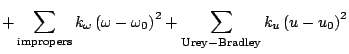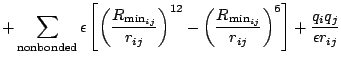(1)

The first term in the energy function accounts for the bond stretches whereis the bond force constant andis the distance from equilibrium that the atom has moved. The second term in the equation accounts for the bond angles whereis the angle force constant andis the angle from equilibrium between 3 bonded atoms. The third term is for the dihedrals (a.k.a. torsion angles) where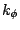is the dihedral force constant,is the multiplicity of the function,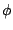is the dihedral angle andis the phase shift. The fourth term accounts for the impropers, that is out of plane bending, where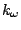is the force constant andis the out of plane angle. The Urey-Bradley component (cross-term accounting for angle bending using 1,3 nonbonded interactions) comprises the fifth term, whereis the respective force constant andis the distance between the 1,3 atoms in the harmonic potential. Nonbonded interactions between pairs of atomsare represented by the last two terms. By definition, the nonbonded forces are only applied to atom pairs separated by at least three bonds. The van Der Waals (VDW) energy is calculated with a standard 12-6 Lennard-Jones potential and the electrostatic energy with a Coulombic potential. In the Lennard-Jones potential above, theterm is not the minimum of the potential, but rather where the Lennard-Jones potential crosses the x-axis (i.e. where the Lennard-Jones potential is zero). In order to run the MD simulations, we need to be sure that our parameter file has all the terms specified in the energy function we are using (i.e. the equation specified above).Next: Developing Topology and Parameter Up: Parameterization Tutorial Previous: Testing out your new
tutorial@ks.uiuc.edu